#6
37
01. 云栖社区>
2. 博客>
3. 正文

## 实时人脸检测

• 树莓派 3 Model B；

• 树莓派摄像头模块（PiCam）。

• OpenCV

• Python 3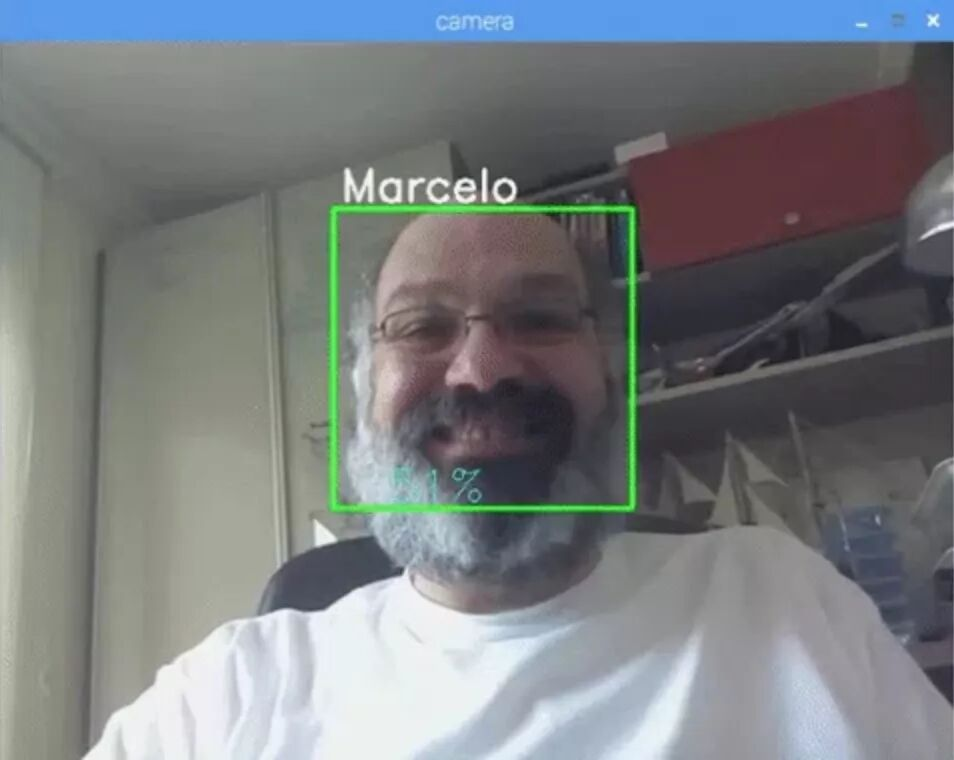1）人脸检测和数据收集；

2）训练识别器；

3）人脸识别。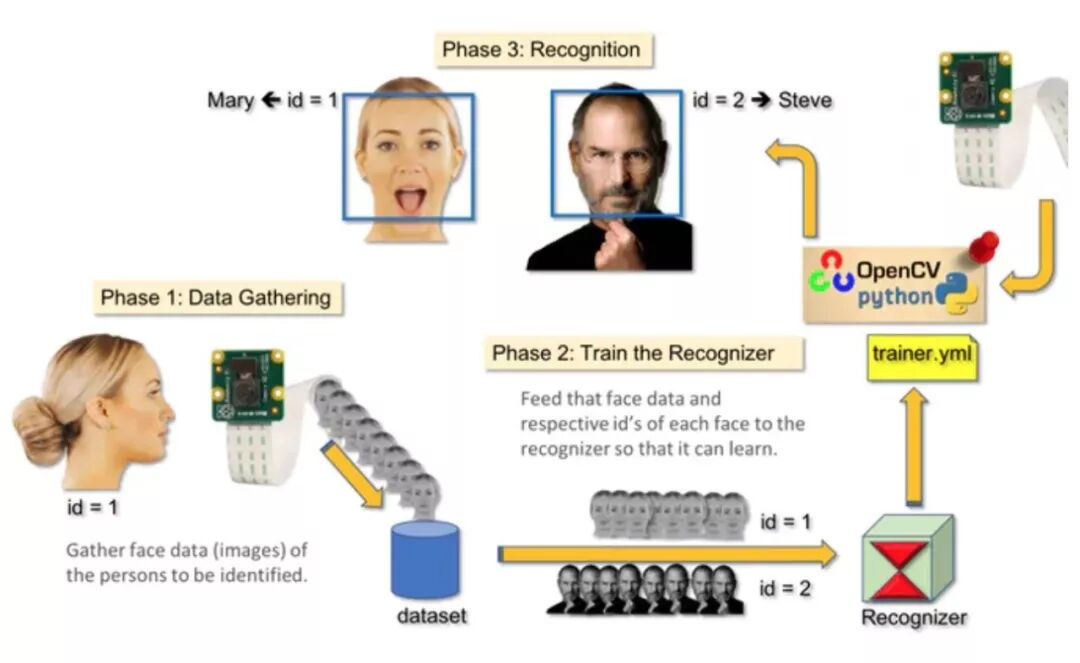• 树莓派 V3：283 RMB（淘宝）

• 500 万像素 1080p 传感器 OV5647 迷你摄像头模块：83 RMB（淘宝）

source ~/.profile

workon cv

(cv) pi@raspberry:~\$

Adrian 希望大家注意 cv Python 虚拟环境是完全独立的，且与 Raspbian Stretch 中包含的默认 Python 版本彼此隔绝。因此，全局站点包目录中的任意 Python 包对于 cv 虚拟环境而言都是不可用的。类似地，cv 站点包中的任意 Python 包对于全局 Python 包安装也都是不可用的。

python

import cv2

cv2.__version__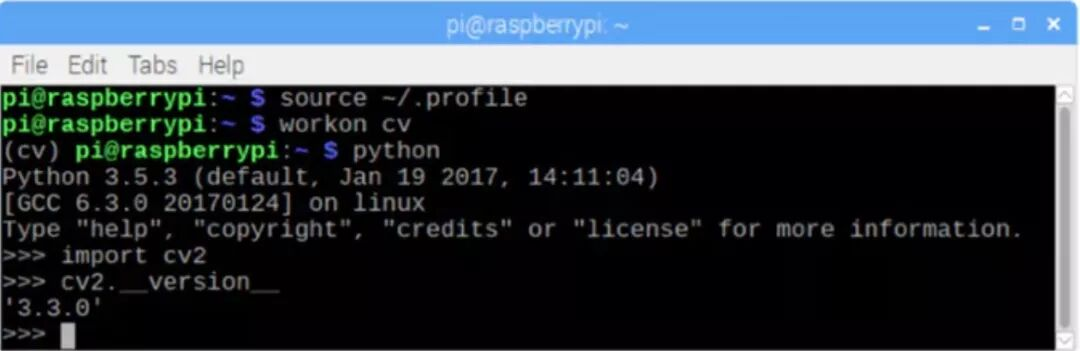import numpy as np
import cv2
cap = cv2.VideoCapture(0)
cap.set(3,640) # set Width
cap.set(4,480) # set Height
while(True):
frame = cv2.flip(frame, -1) # Flip camera vertically
gray = cv2.cvtColor(frame, cv2.COLOR_BGR2GRAY)

cv2.imshow('frame', frame)
cv2.imshow('gray', gray)

k = cv2.waitKey(30) & 0xff
if k == 27: # press 'ESC' to quit
break
cap.release()
cv2.destroyAllWindows()

python simpleCamTest.py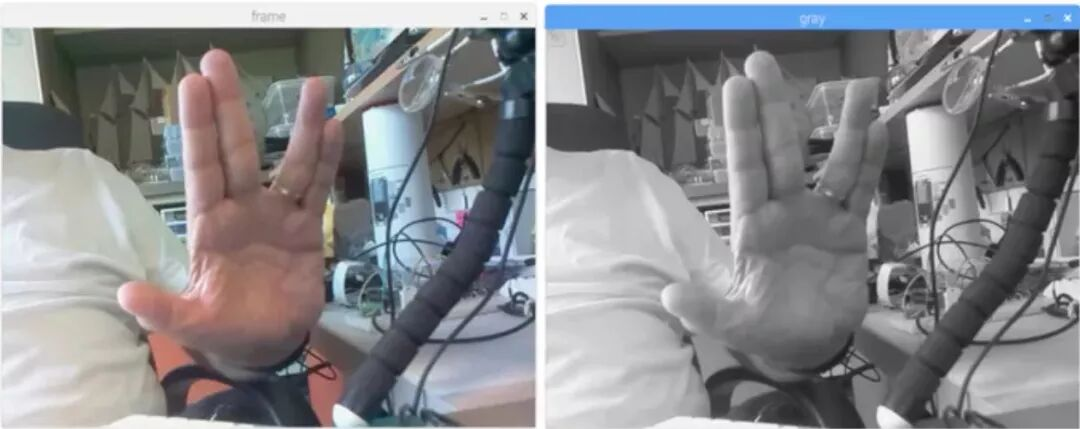import numpy as np
import cv2
cap = cv2.VideoCapture(0)
cap.set(3,640) # set Width
cap.set(4,480) # set Height
while True:
img = cv2.flip(img, -1)
gray = cv2.cvtColor(img, cv2.COLOR_BGR2GRAY)
gray,
scaleFactor=1.2,
minNeighbors=5,
minSize=(20, 20)
)
for (x,y,w,h) in faces:
cv2.rectangle(img,(x,y),(x+w,y+h),(255,0,0),2)
roi_gray = gray[y:y+h, x:x+w]
roi_color = img[y:y+h, x:x+w]
cv2.imshow('video',img)
k = cv2.waitKey(30) & 0xff
if k == 27: # press 'ESC' to quit
break
cap.release()
cv2.destroyAllWindows()

gray,
scaleFactor=1.2,
minNeighbors=5,
minSize=(20, 20)
)

• gray 表示输入 grayscale 图像。

• scaleFactor 表示每个图像缩减的比例大小。

• minNeighbors 表示每个备选矩形框具备的邻近数量。数字越大，假正类越少。

• minSize 表示人脸识别的最小矩形大小。

for (x,y,w,h) in faces:
cv2.rectangle(img,(x,y),(x+w,y+h),(255,0,0),2)
roi_gray = gray[y:y+h, x:x+w]
roi_color = img[y:y+h, x:x+w]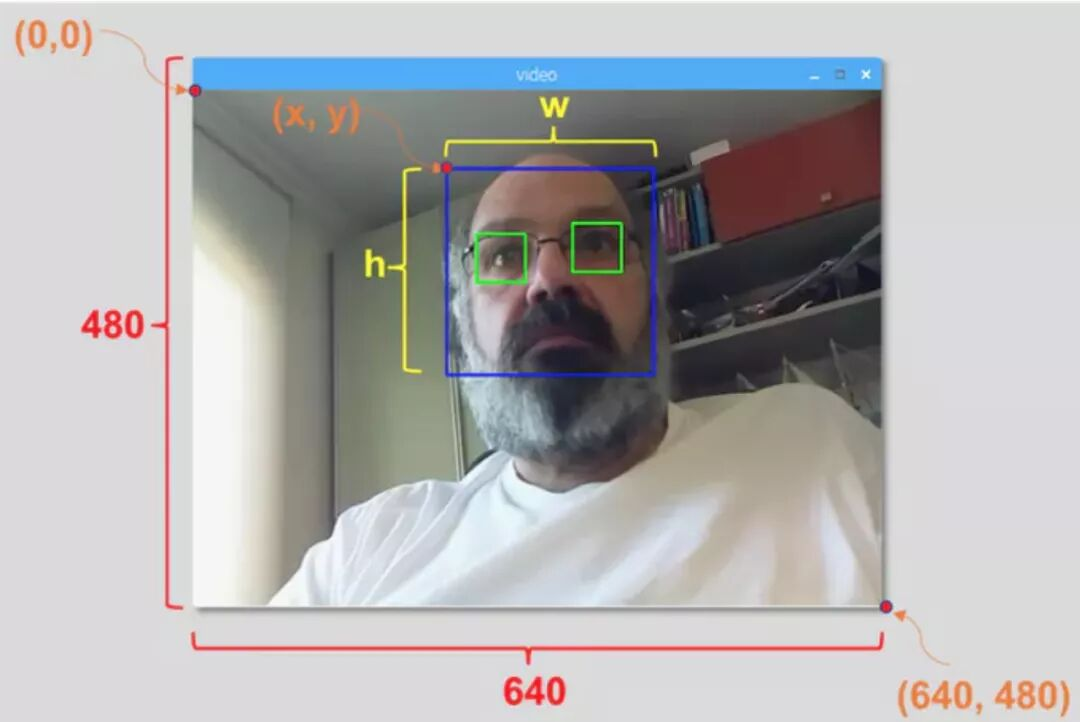python faceDetection.py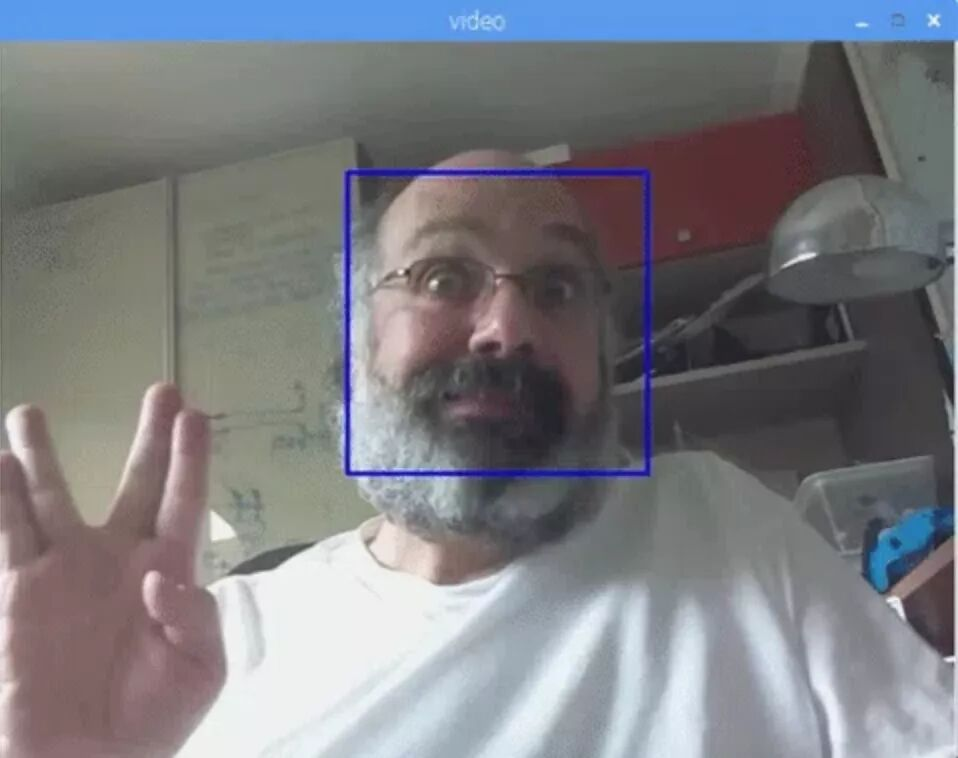• faceEyeDetection.py

• faceSmileDetection.py

• faceSmileEyeDetection.py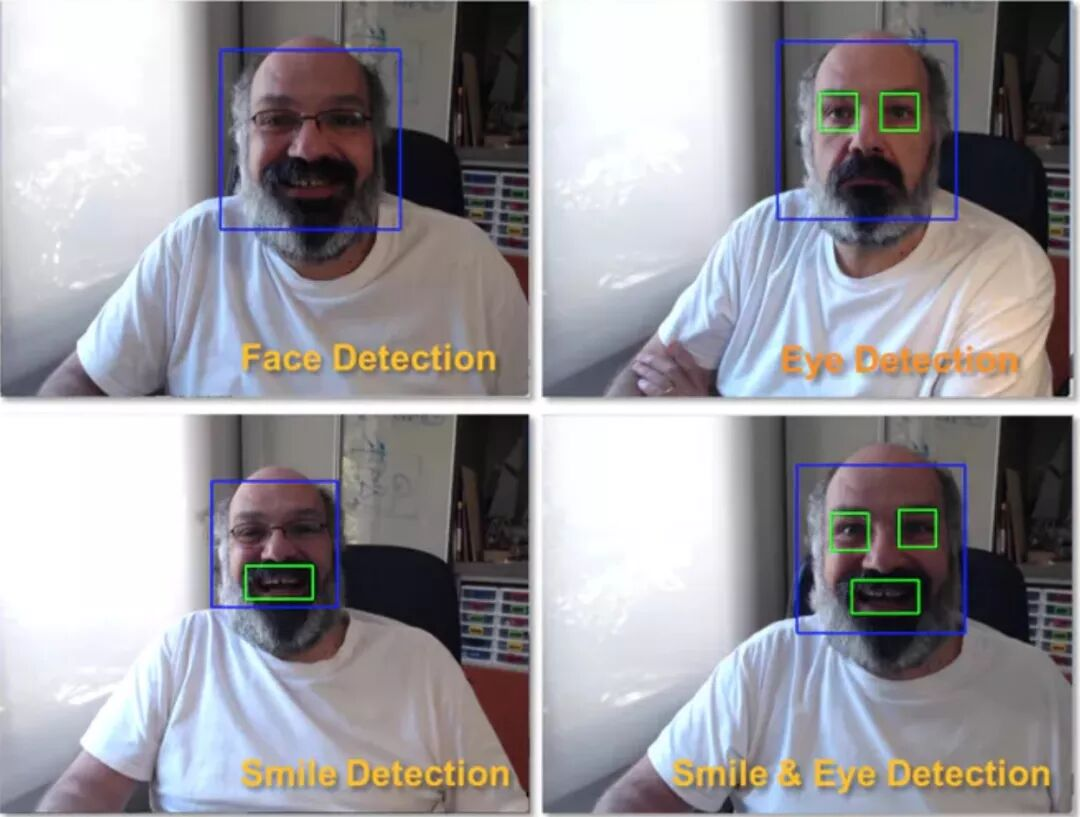• 使用 OpenCV 和 Python 从头实现人脸识别：https://www.superdatascience.com/opencv-face-recognition/

• 理解人脸识别：https://thecodacus.com/category/opencv/face-recognition/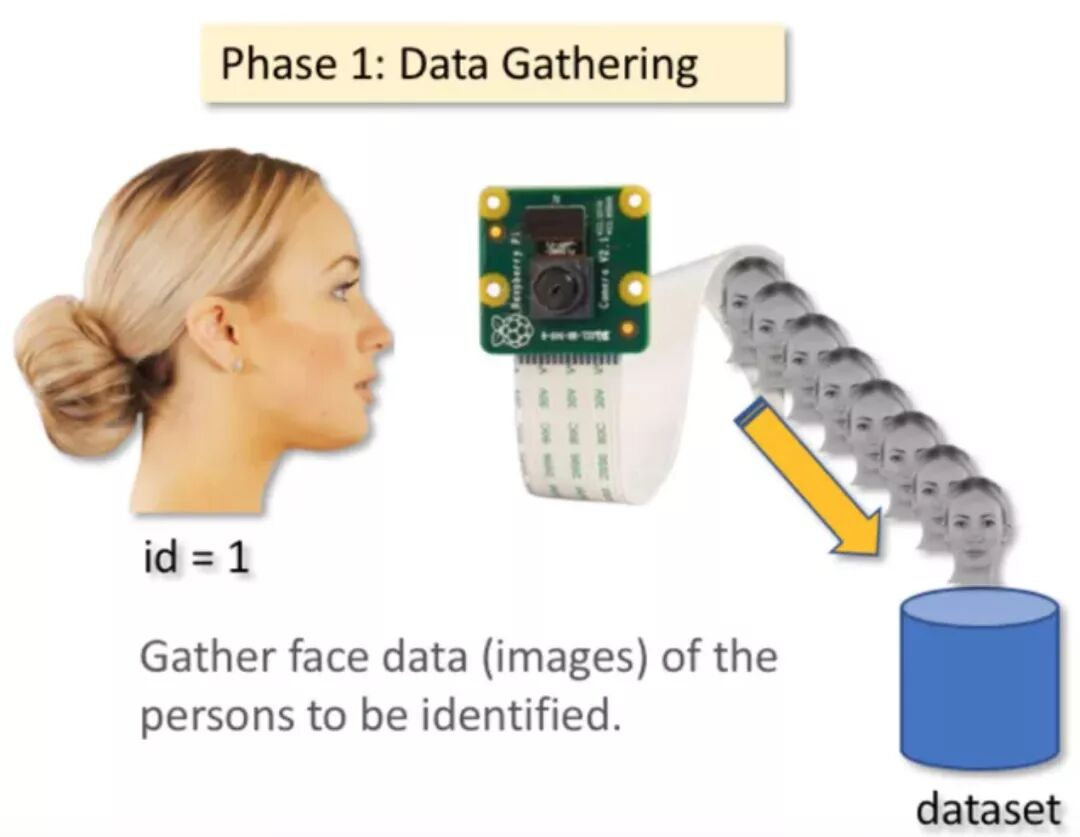mkdir FacialRecognitionProject

mkdir dataset

import cv2
import os
cam = cv2.VideoCapture(0)
cam.set(3, 640) # set video width
cam.set(4, 480) # set video height
# For each person, enter one numeric face id
face_id = input('\n enter user id end press <return> ==>  ')
print("\n [INFO] Initializing face capture. Look the camera and wait ...")
# Initialize individual sampling face count
count = 0
while(True):
img = cv2.flip(img, -1) # flip video image vertically
gray = cv2.cvtColor(img, cv2.COLOR_BGR2GRAY)
faces = face_detector.detectMultiScale(gray, 1.3, 5)
for (x,y,w,h) in faces:
cv2.rectangle(img, (x,y), (x+w,y+h), (255,0,0), 2)
count += 1
# Save the captured image into the datasets folder
cv2.imwrite("dataset/User." + str(face_id) + '.' + str(count) + ".jpg", gray[y:y+h,x:x+w])
cv2.imshow('image', img)
k = cv2.waitKey(100) & 0xff # Press 'ESC' for exiting video
if k == 27:
break
elif count >= 30: # Take 30 face sample and stop video
break
# Do a bit of cleanup
print("\n [INFO] Exiting Program and cleanup stuff")
cam.release()
cv2.destroyAllWindows()

face_id = input('\n enter user id end press  ==>  ')

cv2.imwrite("dataset/User." + str(face_id) + '.' + str(count) + ".jpg", gray[y:y+h,x:x+w])

User.face_id.count.jpg

User.1.4.jpgmkdir trainer

import cv2
import numpy as np
from PIL import Image
import os
# Path for face image database
path = 'dataset'
recognizer = cv2.face.LBPHFaceRecognizer_create()
# function to get the images and label data
def getImagesAndLabels(path):
imagePaths = [os.path.join(path,f) for f in os.listdir(path)]
faceSamples=[]
ids = []
for imagePath in imagePaths:
PIL_img = Image.open(imagePath).convert('L') # convert it to grayscale
img_numpy = np.array(PIL_img,'uint8')
id = int(os.path.split(imagePath)[-1].split("."))
faces = detector.detectMultiScale(img_numpy)
for (x,y,w,h) in faces:
faceSamples.append(img_numpy[y:y+h,x:x+w])
ids.append(id)
return faceSamples,ids
print ("\n [INFO] Training faces. It will take a few seconds. Wait ...")
faces,ids = getImagesAndLabels(path)
recognizer.train(faces, np.array(ids))
# Save the model into trainer/trainer.yml
recognizer.write('trainer/trainer.yml') # recognizer.save() worked on Mac, but not on Pi
# Print the numer of faces trained and end program
print("\n [INFO] {0} faces trained. Exiting Program".format(len(np.unique(ids))))

pip install pillow

recognizer = cv2.face.LBPHFaceRecognizer_create()

recognizer.train(faces, ids)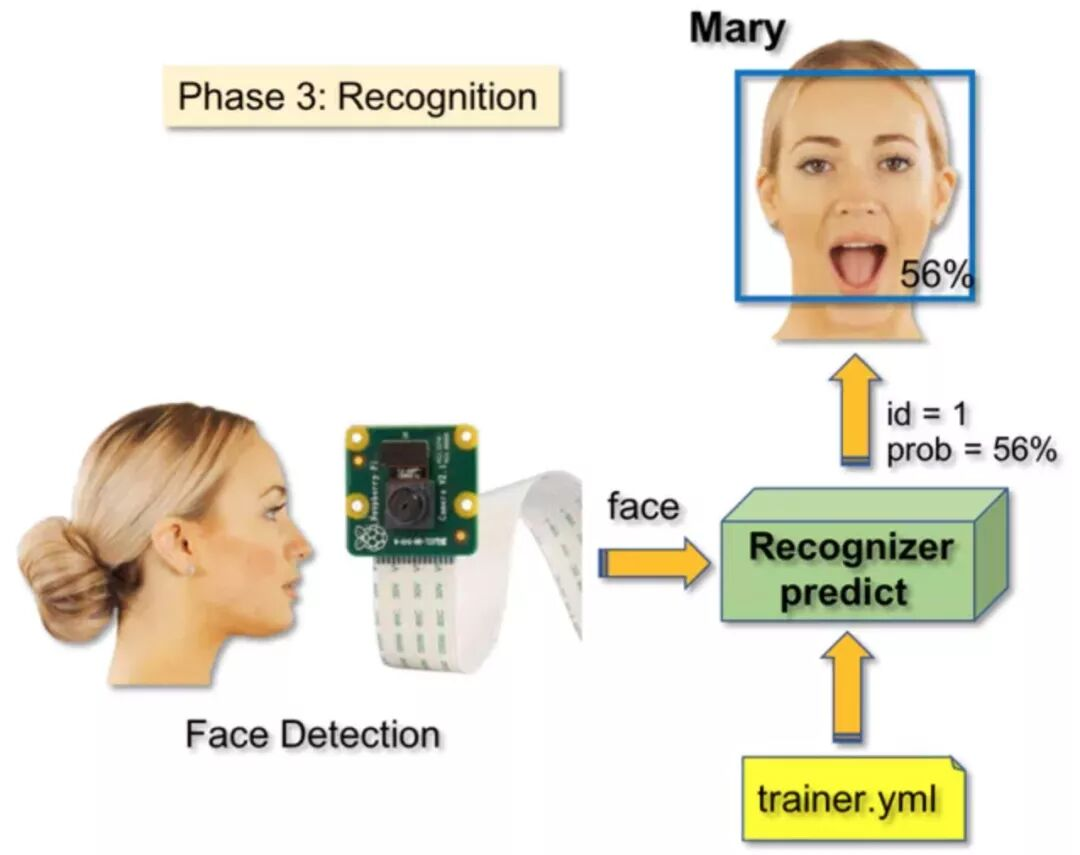import cv2
import numpy as np
import os
recognizer = cv2.face.LBPHFaceRecognizer_create()
font = cv2.FONT_HERSHEY_SIMPLEX
#iniciate id counter
id = 0
# names related to ids: example ==> Marcelo: id=1,  etc
names = ['None', 'Marcelo', 'Paula', 'Ilza', 'Z', 'W']
# Initialize and start realtime video capture
cam = cv2.VideoCapture(0)
cam.set(3, 640) # set video widht
cam.set(4, 480) # set video height
# Define min window size to be recognized as a face
minW = 0.1*cam.get(3)
minH = 0.1*cam.get(4)
while True:
img = cv2.flip(img, -1) # Flip vertically
gray = cv2.cvtColor(img,cv2.COLOR_BGR2GRAY)

gray,
scaleFactor = 1.2,
minNeighbors = 5,
minSize = (int(minW), int(minH)),
)
for(x,y,w,h) in faces:
cv2.rectangle(img, (x,y), (x+w,y+h), (0,255,0), 2)
id, confidence = recognizer.predict(gray[y:y+h,x:x+w])
# Check if confidence is less them 100 ==> "0" is perfect match
if (confidence < 100):
id = names[id]
confidence = "  {0}%".format(round(100 - confidence))
else:
id = "unknown"
confidence = "  {0}%".format(round(100 - confidence))

cv2.putText(img, str(id), (x+5,y-5), font, 1, (255,255,255), 2)
cv2.putText(img, str(confidence), (x+5,y+h-5), font, 1, (255,255,0), 1)

cv2.imshow('camera',img)
k = cv2.waitKey(10) & 0xff # Press 'ESC' for exiting video
if k == 27:
break
# Do a bit of cleanup
print("\n [INFO] Exiting Program and cleanup stuff")
cam.release()
cv2.destroyAllWindows()

names = ['None', 'Marcelo', 'Paula', 'Ilza', 'Z', 'W']

id, confidence = recognizer.predict(gray portion of the face)

recognizer.predict () 将把待分析人脸的已捕捉部分作为一个参数，并返回其可能的所有者，指示其 id 以及识别器与这一匹配相关的置信度。from:https://mp.weixin.qq.com/s?__biz=MzA3MzI4MjgzMw==&mid=2650738591&idx=1&sn=68f8ec3e540eb0d7ca600dc7f52955f9&chksm=871acbe1b06d42f72a05a3441bf4f11960ca121545d5608d6166f998ce1dce68e6b5e1832c9c&mpshare=1&scene=23&srcid=0313fBx9u0yqeVhKxvoK6kXr#rd

+ 关注